Home | | Maths 10th Std | Exercise 8.1: Measures of Dispersion

# Exercise 8.1: Measures of Dispersion

1. Range 2. Mean deviation 3. Quartile deviation 4. Standard deviation 5. Variance 6. Coefficient of Variation (Maths Book back answers and solution for Exercise questions)

Exercise 8.1

1. Find the range and coefficient of range of the following data.

(i) 63, 89, 98, 125, 79, 108, 117, 68

(ii) 43.5, 13.6, 18.9, 38.4, 61.4, 29.8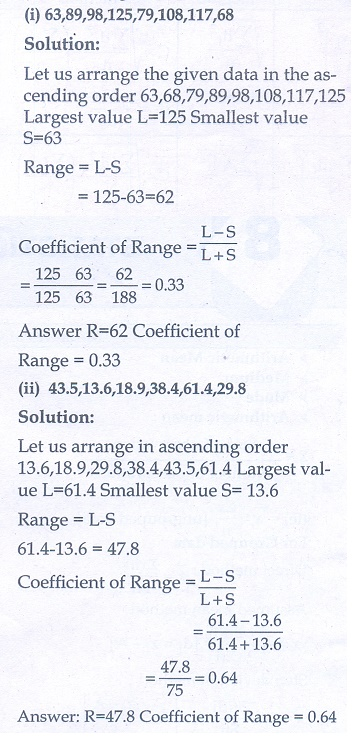2. If the range and the smallest value of a set of data are 36.8 and 13.4 respectively, then find the largest value.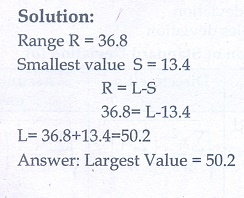3. Calculate the range of the following data.4. A teacher asked the students to complete 60 pages of a record note book. Eight students have completed only 32, 35, 37, 30, 33, 36, 35 and 37 pages. Find the standard deviation of the pages yet to be completed by them.5. Find the variance and standard deviation of the wages of 9 workers given below: ₹310, ₹290, ₹320, ₹280, ₹300, ₹290, ₹320, ₹310, ₹280.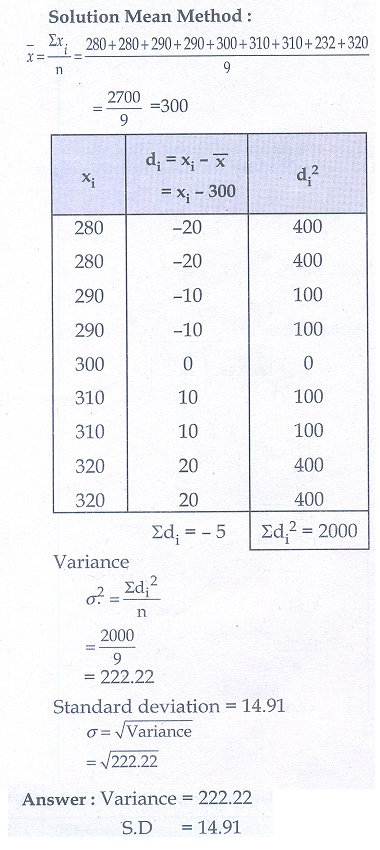6. A wall clock strikes the bell once at 1 o’ clock, 2 times at 2 o’ clock, 3 times at 3 o’ clock and so on. How many times will it strike in a particular day. Find the standard deviation of the number of strikes the bell make a day.7. Find the standard deviation of first 21 natural numbers.8. If the standard deviation of a data is 4.5 and if each value of the data is decreased by 5, then find the new standard deviation.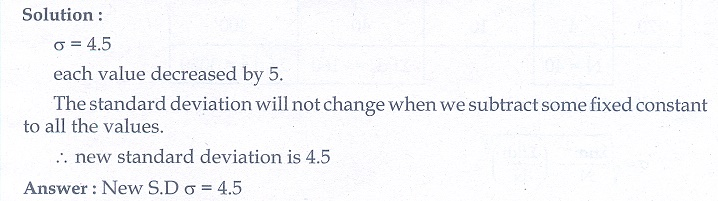9. If the standard deviation of a data is 3.6 and each value of the data is divided by 3, then find the new variance and new standard deviation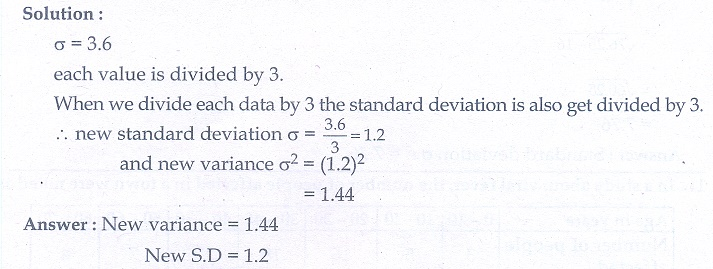10. The rainfall recorded in various places of five districts in a week are given below.Find its standard deviation.11. In a study about viral fever, the number of people affected in a town were noted as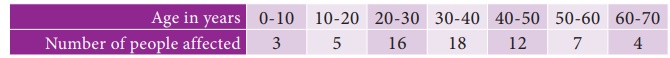Find its standard deviation.12. The measurements of the diameters (in cms) of the plates prepared in a factory are given below. Find its standard deviation.13. The time taken by 50 students to complete a 100 meter race are given below. Find its standard deviation.14. For a group of 100 candidates the mean and standard deviation of their marks were found to be 60 and 15 respectively. Later on it was found that the scores 45 and 72 were wrongly entered as 40 and 27. Find the correct mean and standard deviation.15. The mean and variance of seven observations are 8 and 16 respectively. If five of these are 2, 4, 10, 12 and 14, then find the remaining two observations.1.(i) 62; 0.33 (ii) 47.8; 0.64

2. 50.2

3. 250

4. 2.34

5. 222.22, 14.91

6. 6.9

7. 6.05

8. 4.5

9. 1.44, 1.2

10. 7.76

11. 14.6

12. 6

13. 1.24

14. 60.5, 14.61

15. 6 and 8

Tags : Problem Questions with Answer, Solution | Mathematics , 10th Mathematics : UNIT 8 : Statistics And Probability
Study Material, Lecturing Notes, Assignment, Reference, Wiki description explanation, brief detail
10th Mathematics : UNIT 8 : Statistics And Probability : Exercise 8.1: Measures of Dispersion | Problem Questions with Answer, Solution | Mathematics